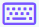Easy
Medium
Hard

## Limit Calculator with steps

Limit calculator helps you find the limit of a function with respect to a variable. It is an online tool that assists you in calculating the value of a function when an input approaches some specific value.Limit calculator with steps shows the step-by-step solution of limits along with a plot and series expansion. It employs all limit rules such as sum, product, quotient, and L'hopital's rule to calculate the exact value.

You can evaluate limits with respect to $$\text{x , y, z , v, u, t}$$ and $$w$$ using this limits calculator.

That’s not it. By using this tool, you can also find,

1. Right-hand limit (+)
2. Left-hand limit (-)
3. Two-sided limit

## How does the limit calculator work?

To evaluate the limit using this limit solver, follow the below steps.

• Enter the function in the given input box.
• Select the concerning variable.
• Enter the limit value.
• Choose the side of the limit. i.e., left, right, or two-sided.
• Hit the Calculate button for the result.
• Use the Reset button to enter new values and the Keypad iconto enter additional values.

You will find the answer below the tool. Click on Show Steps to see the step-by-step solution.

## What is a limit in Calculus?

The limit of a function is the value that f(x) gets closer to as x approaches some number. Limits can be used to define the derivatives, integrals, and continuity by finding the limit of a given function. It is written as:

$$\lim _{x\to a}\:f\left(x\right)=L$$

If f is a real-valued function and a is a real number, then the above expression is read as,

the limit of f of x as x approaches a equals L.## How to find the limit? – With steps

Limits can be applied as numbers, constant values (π, G, k), infinity, etc. Let’s go through a few examples to learn how to calculate limits.

Example - Right-hand Limit

$$\lim _{x\to \:2^+}\frac{\left(x^2+2\right)}{\left(x-1\right)}$$

Solution:

A right-hand limit means the limit of a function as it approaches from the right-hand side.

Step 1: Apply the limit x➜2 to the above function. Put the limit value in place of x.
$$\lim \:_{x\to 2^+}\frac{\left(x^2+2\right)}{\left(x-1\right)}$$

$$=\frac{\left(2^2+2\right)}{\left(2-1\right)}$$

Step 2: Solve the equation to reach a result.

$$=\frac{\left(4+2\right)}{\left(2-1\right)} =\frac{6}{1} =6$$

Step 3: Write the expression with its answer.

$$\lim \:_{x\to \:\:2^+}\frac{\left(x^2+2\right)}{\left(x-1\right)}=6$$

GraphExample - Left-hand Limit

$$\lim _{x\to 3^-}\left(\frac{x^2-3x+4}{5-3x}\right)$$

Solution:

A left-hand limit means the limit of a function as it approaches from the left-hand side.

Step 1: Place the limit value in the function.

$$\lim _{x\to 3^-}\left(\frac{x^2-3x+4}{5-3x}\right)$$

$$=\frac{\left(3^2-3\left(3\right)+4\right)}{\left(5-3\left(3\right)\right)}$$

Step 2: Solve the equation further.

$$=\frac{\left(9-9+4\right)}{\left(5-9\right)}$$

$$=\frac{\left(0+4\right)}{\left(-4\right)} =\frac{4}{-4} =-1$$

Step 3: Write down the function as written below.

$$\lim \:_{x\to \:3^-}\left(\frac{x^2-3x+4}{5-3x}\right)=-1$$

GraphExample - Two-sided Limit

$$\lim _{x\to 5}\left(cos^3\left(x\right)\cdot sin\left(x\right)\right)$$

Solution:

A two-sided limit exists if the limit coming from both directions (positive and negative) is the same. It is the same as limit.

Step 1: Substitute the value of limit in the function.
$$\lim _{x\to 5}\left(cos^3\left(x\right)\cdot sin\left(x\right)\right)$$

$$=cos^3\left(5\right)\cdot \:sin\left(5\right)$$

Step 2: Simplify the equation as we did in previous examples.

$$\lim _{x\to 5}\left(cos^3\left(x\right)\cdot sin\left(x\right)\right)$$

$$=cos^3\left(5\right)\:sin\left(5\right)$$

Step 3: The above equation can be considered as the final answer. However, if you want to solve it further, solve the trigonometric values in the equation.

$$=\frac{1141}{50000}\cdot \:-\frac{23973}{25000} =-\frac{10941}{500000}$$

$$\lim \:\:_{x\to \:\:5}\left(cos^3\left(x\right)\cdot \:\:sin\left(x\right)\right)$$

$$=-0.021882$$

Graph## FAQ’s

Does sin x have a limit?

Sin x has no limit. It is because, as x approaches infinity, the y-value oscillates between 1 and −1.

What is the limit of e to infinity?

The limit of e to the infinity (∞) is e.

What is the limit as e^x approaches 0?

The limit as e^x approaches 0 is 1.

What is the limit as x approaches the infinity of ln(x)?

The limit as x approaches infinity of ln(x) is +. The limit of this natural log can be proved by reductio ad absurdum.

• If x >1ln(x) > 0, the limit must be positive.
• As ln(x2) − ln(x1) = ln(x2/x1). If x2>x1, the difference is positive, so ln(x) is always increasing.
• If lim x→∞ ln(x) = M R, we have ln(x) < M x < eM, but x→∞ so M cannot be in R, and the limit must be +∞.

## References

• What is limit calculus? Study.com | Take Online Courses. Earn College Credit. Research Schools, Degrees & Careers.
• Limit calculator with steps -
• Limits: A graphical approach - concept - calculus video by Brightstorm.

Use android or iOS app of our limit calculator on your mobile